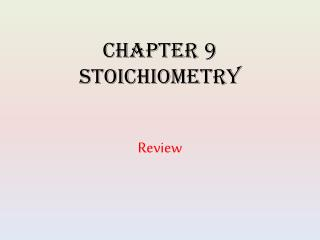DownloadDownload PresentationChapter 9 Stoichiometry

# Chapter 9 Stoichiometry

Télécharger la présentation## Chapter 9 Stoichiometry

- - - - - - - - - - - - - - - - - - - - - - - - - - - E N D - - - - - - - - - - - - - - - - - - - - - - - - - - -
##### Presentation Transcript

1. Chapter 9 Stoichiometry Review

2. Vocabulary • composition stoichiometry • reaction stoichiometry • mole ratio • limiting reactant • excess reactant • percent yield • actual yield • theoretical yield

3. Section 9-1 • Reaction stoichiometry involves the mass relationships between reactants and products in a chemical reaction. • A mole ratio is the conversion factor that relates the amount in moles of any two substances in a chemical reaction. The mole ratio is derived from the balanced equation. • Amount of a substance is expressed in moles, and mass of a substance is expressed using mass units such as grams, kilograms, and milligrams. • Mass and amount of substance are quantities, whereas moles and grams are units. • A balanced chemical equation is necessary to solve any stoichiometric problem.

4. Section 9-2 • In an ideal stoichiometric calculation, the mass or the amount of any reactant or product can be calculated if the balanced chemical equation and the mass or amount of any other reactant or product are known. • Types of Stoichiometry problems: Mole – Mole, Mass – Mole, Mole – Mass, Mass – Mass.

5. Section 9-3 • In actual reactions, the reactants are usually combined in proportions different from the precise proportions required for complete reaction. • The limiting reactant controls the maximum possible amount of product formed. • Given certain quantities of reactants, the quantity of the product is always less than the maximum possible. Percent yield shows the relationship between the theoretical yield and actual yield for the product of a reaction. percent = actual yield x 100 yield theoretical yield

6. Answer the following questions 1. Given the following equation: C3H4(g) + x O2(g) 3CO2(g) + 2H2O(g) a. What is the value of the coefficient x in this equation? b. What is the molar mass of C3H4? c. What is the mole ratio of O2 to H2O in the above equation? d. How many moles are in an 8.0 g sample of C3H4? e. If z mols of C3H4 react, how many moles of CO2 are produced, in terms of z?

7. Review Answers #1. a.4 b. 40.0 g/mol c. 2 mol O2:1 mol H2O d. 0.20 mol e. 3z

8. Review Questions 2. a. What is meant by ideal conditions relative to stoichiometric calculations? b. What function do ideal stoichiometric calculations serve? c. Are actual yields typically larger or smaller than theoretical yields?

9. Review Answers #2. • The limiting reactant is completely converted to product with no losses, as dictated by the ratio of coefficients. b. They determine the theoretical yield of the products of the reaction. c. smaller

10. Review Questions 3. Assume the reaction represented by the following equation goes all the way to completion: N2 + 3H2 2NH3 a. If 6 mol of H2 are consumed, how many moles of NH3are produced? b. How many grams are in a sample of NH3 that contains 3.0 x1023 molecules? c. If 0.1 mol of N2 combine with H2, what must be true about the quantity of H2 for N2 to be the limiting reactant?

11. Review Answers #3. a. 4 mol b. 8.5 g c. At least 0.3 mol of H2 must be provided.

12. Review Questions 4. If a reaction’s theoretical yield is 8.0 g and the actual yield is 6.0 g, what is the percentage yield?

14. Review Questions 5. Joseph Priestley generated oxygen gas by strongly heating mercury(II) oxide according to the following equation: 2HgO(s)2Hg(l) + O2(g) a. If 15.0 g HgO decompose, how many moles of HgO does this represent? b. How many moles of O2 are theoretically produced? c. How many grams of O2 is this? d. If the percentage yield is 95.0%, how many grams of O2are actually collected?

16. Review Questions 6. Copper reacts with silver nitrate through single replacement. a. If 2.25 g of silver are produced from the reaction, how many moles of copper(II) nitrate are produced? b. How many moles of each reactant are required to complete this same reaction?

17. Review Answers #6 Cu + 2 AgNO3 2 Ag + Cu(NO3)2 a. 0.0104 mol Cu(NO3)2 b. 0.0104 mol Cu, 0.0209 mol AgNO3

18. Review Questions 7. Sulfuric acid reacts with aluminum hydroxide by double replacement. a. If 30.0 g of sulfuric acid react with 25.0 g of aluminum hydroxide, identify the limiting reactant. b. Determine the mass of excess reactant remaining. c. Determine the mass of each product formed. Assume 90% yield.

19. Review Answers #7 3H2SO4 + 2Al(OH)3 Al2(SO4)3 + 6HOH a. H2SO4 is the limiting reactant. b. 9.05 g excess Al(OH)3 c. 34.9 g Al2(SO4)3 11.0 g H2O

20. Review Questions • Coal gasification is a process that converts coal into methane gas. 2C(s) + 2H2O(l) .→ CH4(g) + CO2(g) a. If this reaction has a percentage yield of 85.0%, what mass of methane can be obtained from 1250 g of carbon? b. If the percentage yield for the coal gasification process is increased to 95%, what mass of methane can be obtained from 2750 g of carbon?